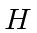## A Complete Set of Mutually Commuting Operators

If an operator commutes with, we can make simultaneous eigenfunctions of energy and that operator. This is an important tool both for solving the problem and for labeling the eigenfunctions.

A complete set of mutually commuting operators will allow us to define a state in terms of the quantum numbers of those operators. Usually, we will need one quantum number for each degree of freedom in the problem.

For example, the Hydrogen atom in three dimensions has 3 coordinates for the internal problem, (the vector displacement between the proton and the electron). We will need three quantum numbers to describe the state. We will use an energy index, and two angular momentum quantum numbers to describe Hydrogen states. The operators will all commute with each other. The Hydrogen atom also has 3 coordinates for the position of the atom. We will might use,andto describe that state. The operators commute with each other.

If we also consider the spin of the electron in the Hydrogen atom, we find that we need to add one more commuting operator to label the states and to compute the energies accurately. If we also add the spin of the proton to the problem, the we need still one more quantum number to describe the state.

If it is possible, identifying the commuting operators to be used before solving the problem will usually save time.

Jim Branson 2013-04-22